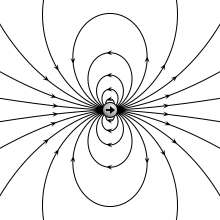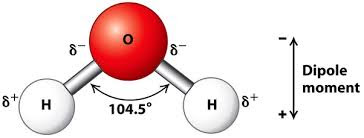# What is Electric Dipole , Dipole Moment and Dipole Field ? | cbse24.com

### Electric Dipole:

A system consisting of positive and a negative charge of equal magnitude q, separated by a distance d is called an electric dipole.Electric Dipole

### Dipole moment:

It measures the strength of an electric dipole. The dipole moment of an electric dipole is a vector whose magnitude is either charge time the separation between the two opposite charges and the direction is along the dipole axis from the negative to the positive charge.Animation showing the electric field of an electric dipole.

As shown in figure consider an electric dipole consisting of charge +q and -q and separated by distance 2a .the line joining the charges is called dipole axis.

Dipole moment=Either charge  X  a vector drawn from negative to the positive charge

Thus the dipole moment is a vector quantity. its direction is along the dipole axis from -q  to +q and its magnitude is

• The SI unit of dipole moment is Colomb meter (C m)
• A commonly used unit in atomic physics and chemistry is the debye (D) 1 D = 10−18 statC*cm
1D =3.335×1030 Cc C * meter
• The direction of dipole moment is from -q to +q

### Examples of electric dipole:

The dipole is common in nature. In molecules like H2O, HCl, C2H5OH, etc, the center of positive charge does not fall exactly over the center of negative charges. Such molecules are electric dipoles. They have a permanent dipole moment.The dipole moment of water

### Dipole Field:

The electric field produced by an electric dipole is called a dipole field. This can be determined by using (a) the formula for the field of a point charge and (b) the principle of superpositionThe electric field at the equatorial point at dipole and direction of resultantThe electric field at an axial point of a dipole and direction of resultant

Variation of dipole field with distance: the total charge of an electric dipole is zero.but the electric field of an electric dipole is not zero. This is because the charges +q and -q are separated by some distance, so the electric fields due to them when added so not exactly cancel out. However, at distances much larger than the dipole size(r>>2a), then fields of +q and -q nearly cancel out. Hence we expect a dipole field to fall off, at a larger distance, faster than  , typical of the field due to a single charge. In fact, a dipole field at larger distances falls as  .

1.2.